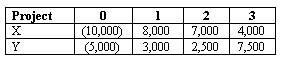### CFA Practice Question

There are 443 practice questions for this topic.

### CFA Practice Question

Beaumont Bearings is analyzing two mutually exclusive projects with the following cash flows. Its cost of capital is 9%.The NPV of projects X and Y are:
A. X: 6,320; Y: 5,648
B. X: 9,000; Y: 8,000
C. X: 7,339; Y: 2,752
Explanation: NPVX = -10,000 + 8,000/(1.09)1 + 7,000/(1.09)2 + 4,000/(1.09)3 = 6,320
NPVY = -5,000 + 3,000/(1.09)1 + 2,500/(1.09)2 + 7,500/(1.09)3 = 5,648